# How to make Pattern Shift/Size per side?

Hi sir,
As the picture 1 , When I find some of location out of rule (space)
as this sample , rule is 20um , only edge A to U and J to N is out of rule.

I want to make the space(gap) as picture 2 ....no modify on the other side of pattern
just reduce (edit) partial of edge A /U / J/N to match with rule.
here was my simple code , and It can't make it as what I want , do you have any idea for that?

``````psv=input(30,8)
ruleP=3000
ruleSpace=22
big_psv=psv.without_area(0,ruleP.um)
small_psv=psv.without_area(0,ruleP.um)
big_psv.isolated(ruleSpace.um,projection).output(151,400)
psv_not=input(151,400)
psv.not(psv_not.sized(1.um)).output(151,500)
``````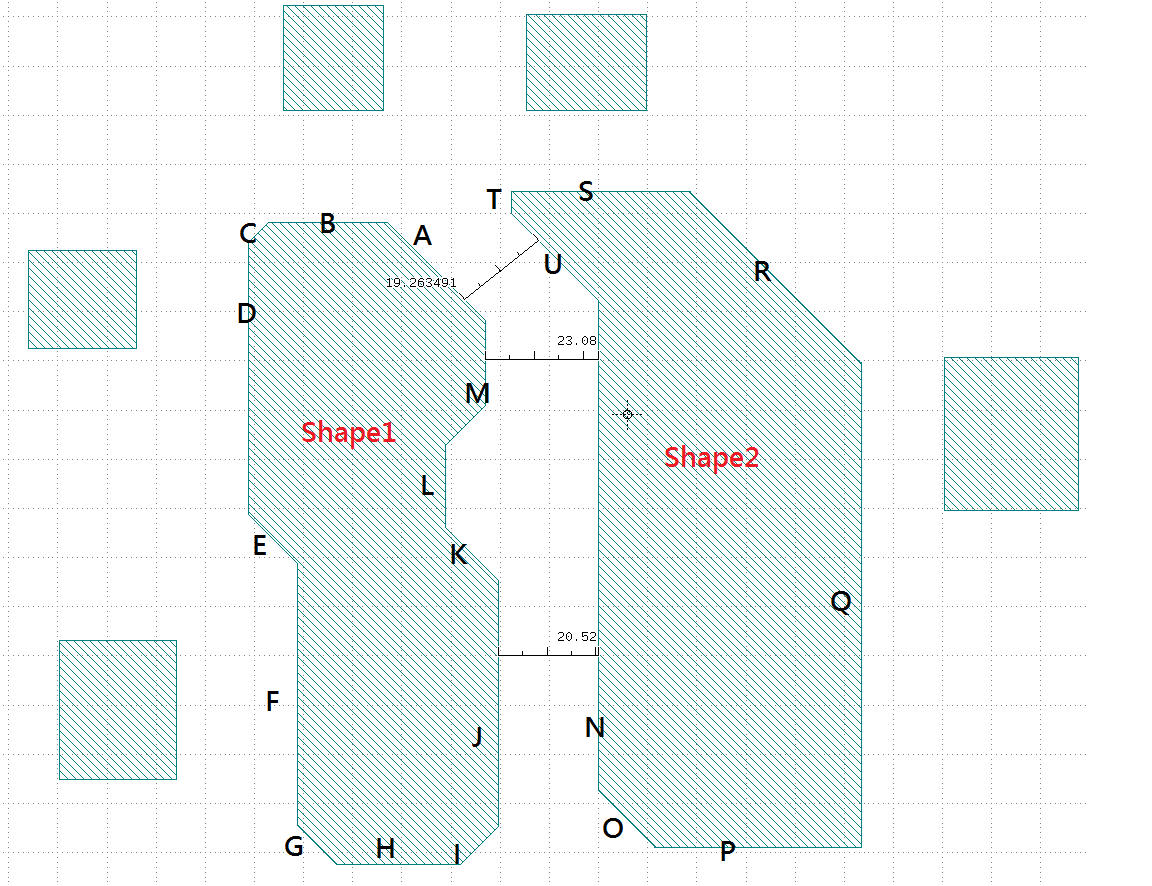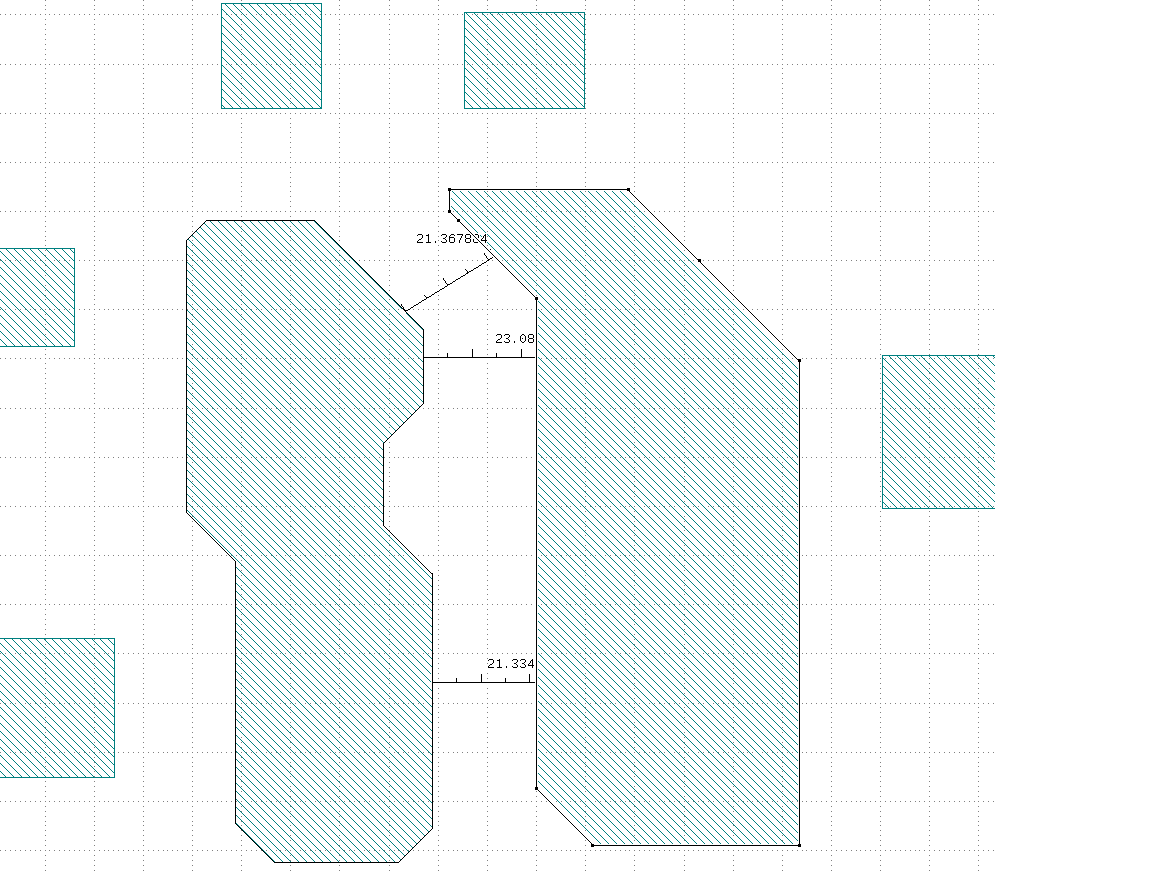• edited September 4

Hi jiunnweiyeh

I found this is very difficult to be achieve by DRC function,

Here's an example of filtering out rule violated edges, shift them and stitch them back to from a shape using Ruby API.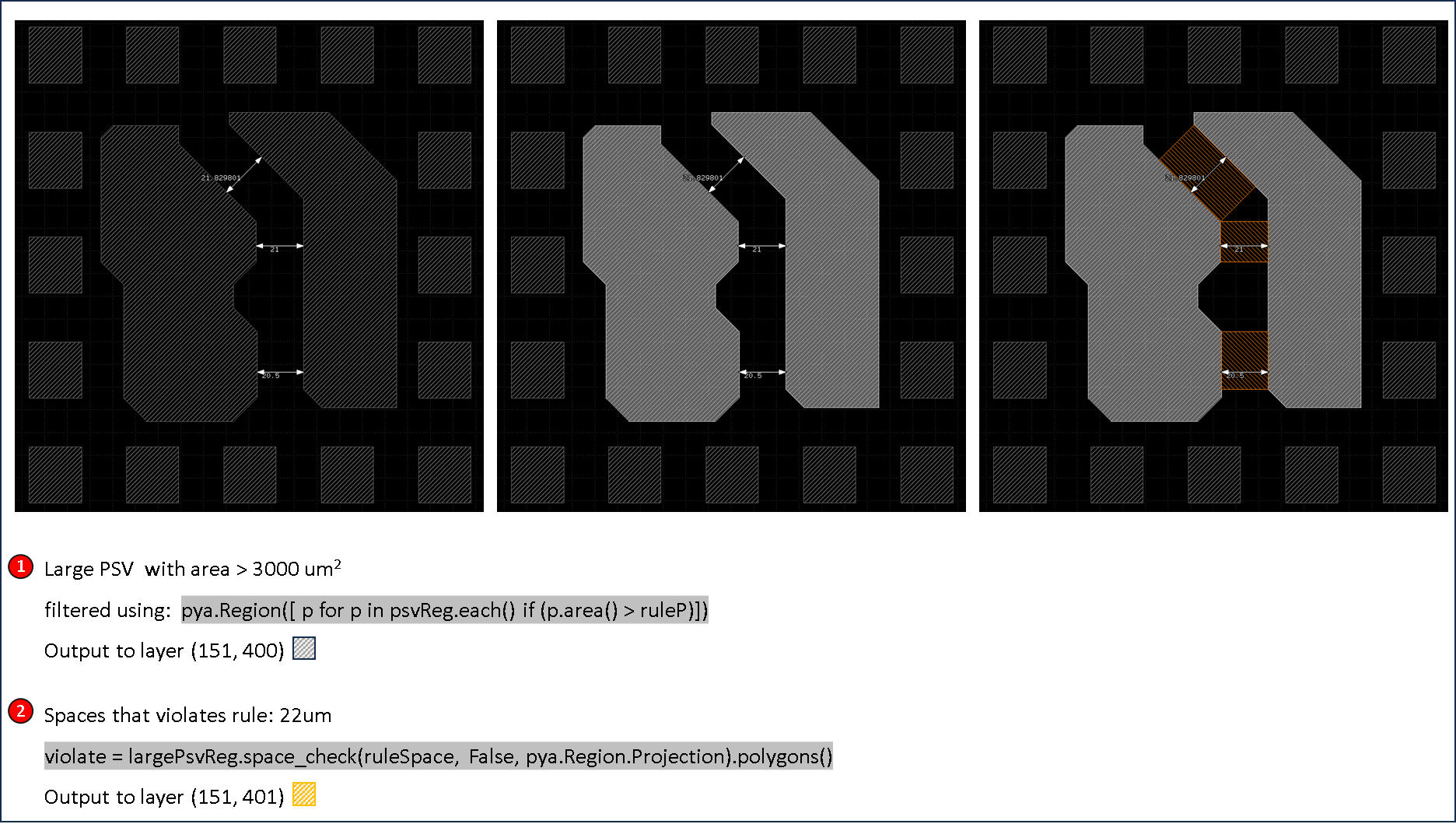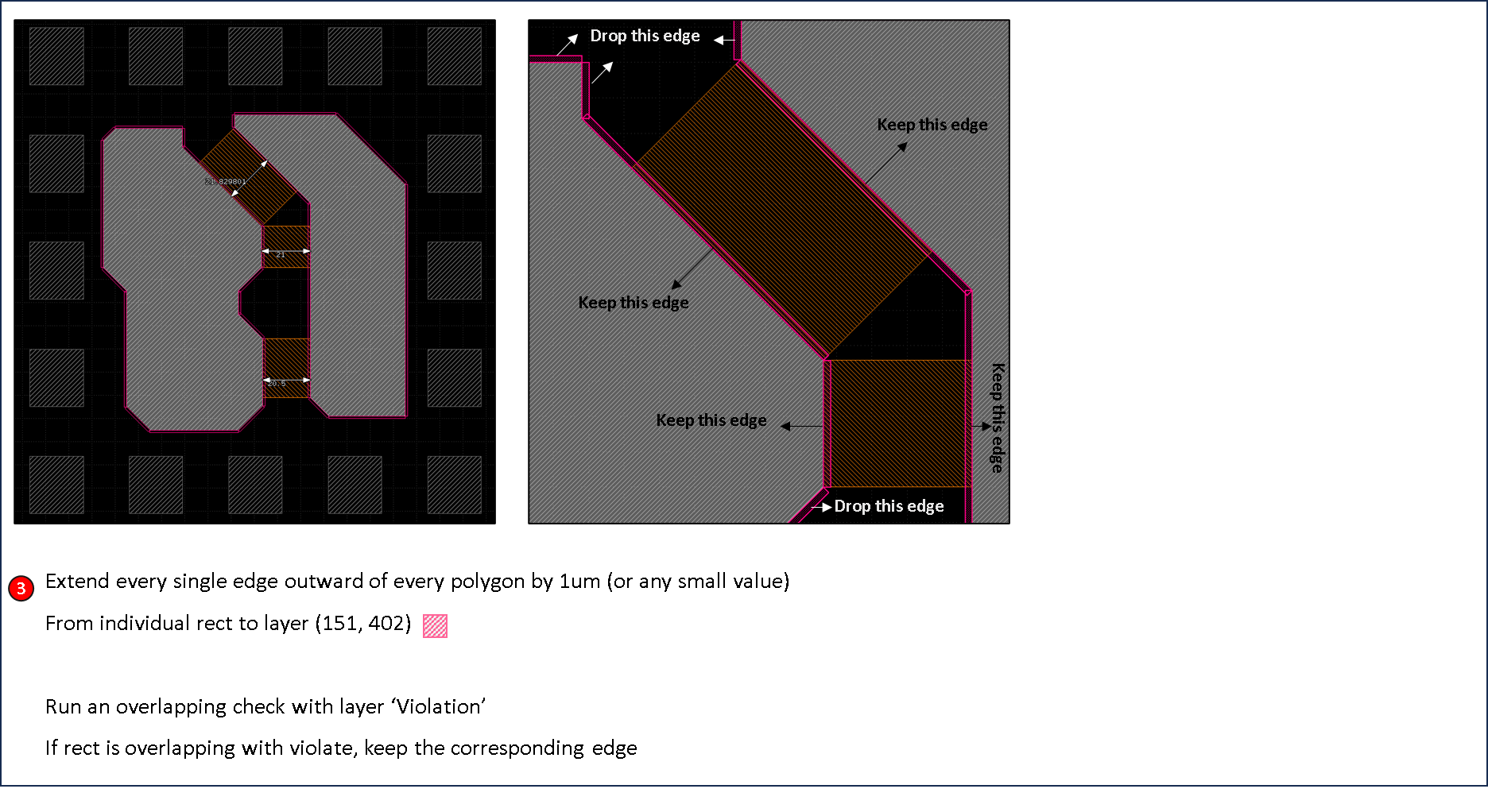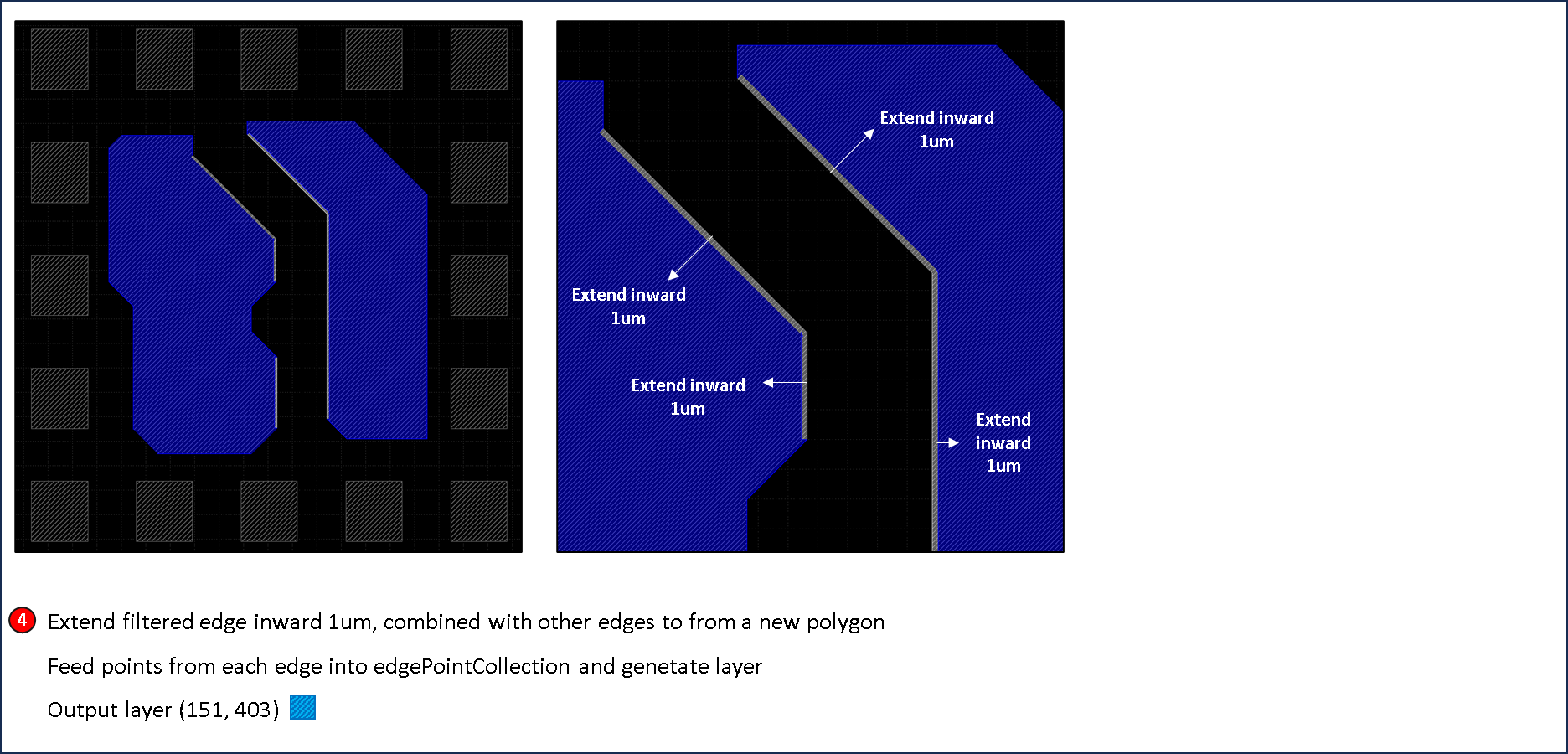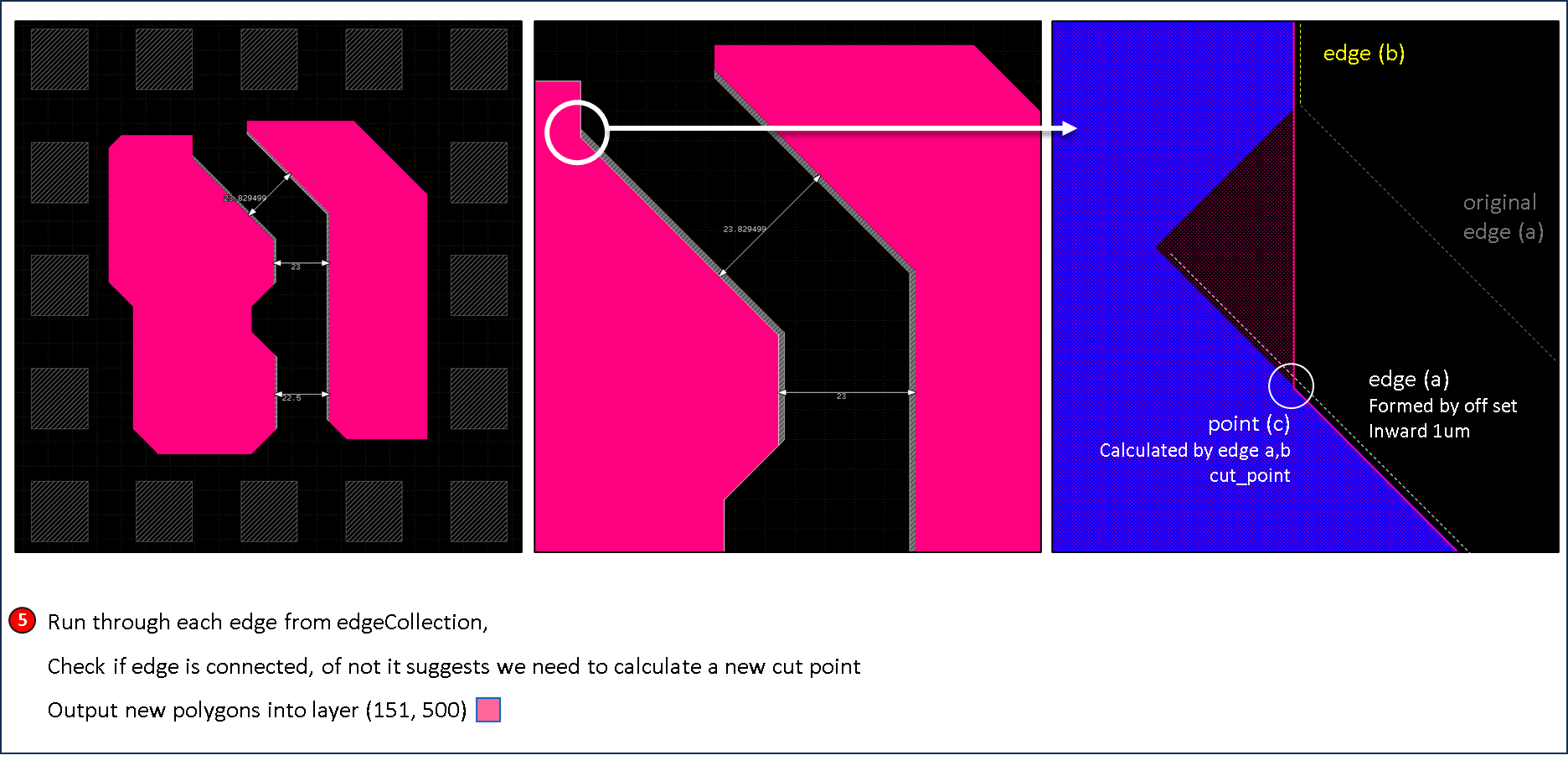``````\$mainWindow = RBA::Application::instance::main_window
\$layoutView = \$mainWindow::current_view
\$cellView   = \$layoutView::active_cellview
\$layout     = \$cellView::layout
\$cell       = \$cellView::cell
\$unit       = \$layout::dbu
\$um         = 1/\$unit

def input(l, d)
result = RBA::Region::new()
\$cell.each_shape(\$layout.layer(l, d))do |shape|
result.insert(shape.polygon)
end
return result
end

def output(l, d, region)
\$cell.clear(\$layout.layer(l, d))
\$cell.shapes(\$layout.layer(l, d)).insert(region)
end

ruleP                 = 3000 * um * um
ruleSpace             =   22 * um
psvReg                = input(30, 8)
largePsvReg           = RBA::Region::new(psvReg.each.to_a.select { |poly| poly.area > ruleP })      # step -1 filter large shapes
violate               = largePsvReg.space_check(ruleSpace, false, RBA::Region::Projection).polygons # step -2 check violation

newEdgeCollectionReg  = RBA::Region::new()
edgePolyCollectionReg = RBA::Region::new()
edgeOutExtReg         = RBA::Region::new()

largePsvReg.each do |poly|
edgeCollection      = []
newEdgeCollection   = []
edgePointCollection = []

poly.each_edge do |edge|

extOutEdge     = edge.shifted(1 * \$um)
extEdgePoly    = RBA::Polygon::new([edge.p1, edge.p2, extOutEdge.p2, extOutEdge.p1, edge.p1]) # step -3 forms extended edges
extEdgeReg     = RBA::Region::new(extEdgePoly)
edgeOutExtReg += RBA::Region::new(extEdgePoly)

if extEdgeReg.overlapping(violate).count > 0     # step -3 filter edges by overlapping check
extInEdge = edge.shifted( - 1 * \$um)         # step -4 extend filtered edge inward 1um and from new polygons
edgeCollection.append(extInEdge)
edgePointCollection.append(extInEdge.p1)
edgePointCollection.append(extInEdge.p2)

else
edgeCollection.append(edge)                  # step -4 extend filtered edge inward 1um and from new polygons
edgePointCollection.append(edge.p1)
edgePointCollection.append(edge.p2)
end

end

for i in -1..(edgeCollection.length - 2) do
a = edgeCollection[i]
b = edgeCollection[i + 1]
c = a.p2

if not(a.p2 == b.p1)                             # step -5 check if edges is connected, of not calculate new cut point
c = a.cut_point(b)
end

if i == 0
newEdgeCollection.append(a.p1)
newEdgeCollection.append(c)
newEdgeCollection.append(b.p1)
else
newEdgeCollection.append(c)
end

end

newEdgeCollectionReg  += RBA::Region::new(RBA::Polygon::new(newEdgeCollection))
edgePolyCollectionReg += RBA::Region::new(RBA::Polygon::new(edgePointCollection))

end

output(151, 400, largePsvReg)
output(151, 401, violate)
output(151, 402, edgeOutExtReg)
output(151, 403, edgePolyCollectionReg)
output(151, 500, newEdgeCollectionReg)
``````
• @RawrRanger Thanks for your help , I will try it.

• Hi jiunnweiyeh,

for demostration purpose, previous examples includeds several layers that is not neccessary, here's an condensed version without generating those layers.

``````\$mainWindow = RBA::Application::instance::main_window
\$layoutView = \$mainWindow::current_view
\$cellView   = \$layoutView::active_cellview
\$layout     = \$cellView::layout
\$cell       = \$cellView::cell
\$unit       = \$layout::dbu
\$um         = 1/\$unit

def input(l, d)
return RBA::Region::new(\$cell.each_shape(\$layout.layer(l, d)).collect {|shape| shape.polygon}.to_a)
end

def output(l, d, region)
\$cell.clear(\$layout.layer(l, d))
\$cell.shapes(\$layout.layer(l, d)).insert(region)
end

def mergeEdges(edges)
result = []
for i in -1..(edges.length - 2) do
a, b = edges[i], edges[i + 1]
result.append( (a.p2 == b.p1) ? a.p2 : a.cut_point(b) )
end
return result
end

ruleP                 = 3000 * \$um * \$um
ruleSpace             =   22 * \$um
psvReg                = input(30, 8)
largePsvReg           = RBA::Region::new(psvReg.each.to_a.select { |poly| poly.area > ruleP })      # step -1 filter large shapes
violate               = largePsvReg.space_check(ruleSpace, false, RBA::Region::Projection).polygons # step -2 check violation
newEdgeCollectionReg  = RBA::Region::new()

largePsvReg.each do |poly|
edgeCollection = []
poly.each_edge do |edge|
extOutEdge = edge.shifted(1 * \$um)
extEdgeReg = RBA::Region::new(RBA::Polygon::new([edge.p1, edge.p2, extOutEdge.p2, extOutEdge.p1, edge.p1]))
edgeCollection.append(extEdgeReg.overlapping(violate).count > 0 ? edge.shifted( - 1 * \$um) : edge) # step -3/4 filter edges by overlapping and extend filtered edge inward 1um
end
newEdgeCollectionReg  += RBA::Region::new(RBA::Polygon::new(mergeEdges(edgeCollection))) # step -5 check if edges is connected, of not calculate new cut point
end

output(151, 400, largePsvReg)
output(151, 401, violate)
output(151, 500, newEdgeCollectionReg)
``````